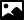## Data Category ihm_geometric_object_distance_restraint

### GeneralCategory name
ihm_geometric_object_distance_restraint
Required in PDB entries
no
Category group membership
ihm_group
Used in current PDB entries
No

### Category Relationship DiagramsView full category relationship diagram including all dictionary data categories

### Category Description```               Data items in the IHM_GEOMETRIC_OBJECT_DISTANCE_RESTRAINT category records the
details of distance restraints involving geometric objects.

If the geometric object involved is a plane, then the distance
is along the normal following the right-hand rule.
So for the xy plane, distance is along the z axis in the positive
direction, 'above' the plane such that negative distances
corresponded to positions below the plane.
```

#### Category Example

```
loop_
_ihm_geometric_object_distance_restraint.id
_ihm_geometric_object_distance_restraint.object_id
_ihm_geometric_object_distance_restraint.feature_id
_ihm_geometric_object_distance_restraint.object_characteristic
_ihm_geometric_object_distance_restraint.restraint_type
_ihm_geometric_object_distance_restraint.harmonic_force_constant
_ihm_geometric_object_distance_restraint.dataset_list_id
1   1    21    'inner surface'    'harmonic'  1.0  1
2   1    22    'inner surface'    'harmonic'  1.0  1
3   1    23    'inner surface'    'harmonic'  1.0  1
4   1    24    'inner surface'    'harmonic'  1.0  1
# ...  abbreviated ...```